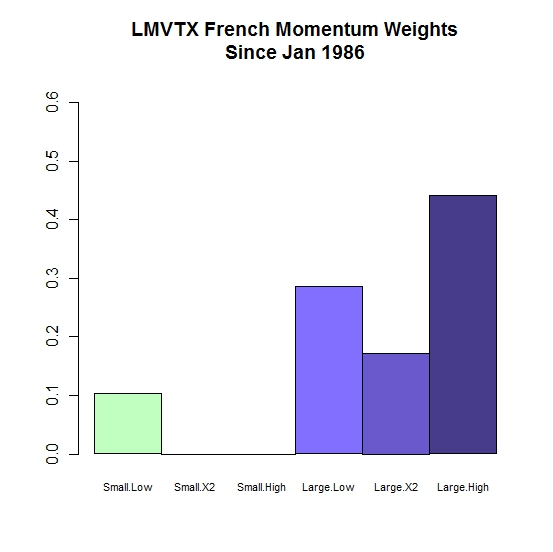Want to share your content on R-bloggers? click here if you have a blog, or here if you don't.

After reading the fine article Style Analysis from Systematic Investor and What we can learn from Bill Miller and the Legg Mason Value Trust from Asymmetric Investment Returns, I thought I should combine the two in R with the FactorAnalytics package.  Let’s explore the Legg Mason Value Trust run by Bill Miller to get some insight into the source of his returns over many years by using the Ken French momentum by size data set as factors.From TimelyPortfolioFrom TimelyPortfolioFrom TimelyPortfolio

`#use Ken French momentum style indexes for style analysis#http://mba.tuck.dartmouth.edu/pages/faculty/ken.french/ftp/6_Portfolios_ME_Prior_12_2.zip   require(PerformanceAnalytics)require(FactorAnalytics)require(quantmod)   my.url="http://mba.tuck.dartmouth.edu/pages/faculty/ken.french/ftp/6_Portfolios_ME_Prior_12_2.zip"my.tempfile<-paste(tempdir(),"\\frenchmomentum.zip",sep="")my.usefile<-paste(tempdir(),"\\6_Portfolios_ME_Prior_12_2.txt",sep="")download.file(my.url, my.tempfile, method="auto", 	quiet = FALSE, mode = "wb",cacheOK = TRUE)unzip(my.tempfile,exdir=tempdir(),junkpath=TRUE)#read space delimited text file extracted from zipfrench_momentum <- read.table(file=my.usefile,	header = TRUE, sep = "",	as.is = TRUE,	skip = 12, nrows=1017)colnames(french_momentum) <- c(paste("Small",	colnames(french_momentum)[1:3],sep="."),	paste("Large",colnames(french_momentum)[1:3],sep="."))   #get dates ready for xts indexdatestoformat <- rownames(french_momentum)datestoformat <- paste(substr(datestoformat,1,4),	substr(datestoformat,5,7),"01",sep="-")   #get xts for analysisfrench_momentum_xts <- as.xts(french_momentum[,1:6],	order.by=as.Date(datestoformat))   french_momentum_xts <- french_momentum_xts/100   #get price series from monthly returnsfrench_price<-as.xts(	apply(1+coredata(french_momentum_xts[,1:6]),MARGIN=2,cumprod),	index(french_momentum_xts))#check data for reasonabilityplot.zoo(french_price,log="y")   #for this example let's use Bill Miller's fundgetSymbols("LMVTX",from="1896-01-01", to=Sys.Date(), adjust=TRUE)LMVTX <- to.monthly(LMVTX)index(LMVTX) <- as.Date(format(as.Date(index(LMVTX)),"%Y-%m-01"))LMVTX.roc <- ROC(LMVTX[,4],type="discrete",n=1)   perfComp <- na.omit(merge(LMVTX.roc,french_momentum_xts))   chart.RollingStyle(perfComp[,1],perfComp[,2:NCOL(perfComp)],	width=36,	colorset=c("darkseagreen1","darkseagreen3","darkseagreen4","slateblue1","slateblue3","slateblue4"),	main="LMVTX Rolling 36mo French Momentum Weights")#could use the packaged chart.Style but does not allow the#flexibility I would like#chart.Style(perfComp[,1],perfComp[,2:NCOL(perfComp)],#	colorset=c("darkseagreen1","darkseagreen3","darkseagreen4","slateblue1","slateblue3","slateblue4"),#	main="LMVTX French Momentum Weights")   #get weights for the cumulative periodstyle.weight <- as.matrix(style.fit(perfComp[,1],	perfComp[,2:NCOL(perfComp)])\$weights)barplot(style.weight,beside=TRUE,ylim=c(0,max(style.weight)+0.2),	names.arg=rownames(style.weight),cex.names=0.7,	col=c("darkseagreen1","darkseagreen3","darkseagreen4",		"slateblue1","slateblue3","slateblue4"),	main=paste("LMVTX French Momentum Weights	Since ",format(index(LMVTX),"%b %Y"),sep=""))   #look at total R to determine goodness of fitstyle.R <- style.fit(perfComp[,1],	perfComp[,2:NCOL(perfComp)])\$R.squared     styleR <- function(x) {	as.numeric(style.fit(R.fund=x[,1,drop=FALSE],R.style=x[,2:NCOL(x),drop=FALSE],method="constrained",selection="none",leverage=FALSE)\$R.squared)}#convert to matrix since I get#error "The data cannot be converted into a time series."#when I use xts as datastyle.RollingR <- as.xts(rollapply(data=as.matrix(perfComp),	width=12,FUN=styleR,by.column=FALSE,by=1),	order.by=index(perfComp)[12:NROW(perfComp)])chart.TimeSeries(style.RollingR,ylab="Rolling 12-mo R",	main=paste("LMVTX Rolling R versus French Momentum	Since ",format(index(LMVTX),"%b %Y"),sep=""))abline(h=style.R,col="indianred")text(x=1,y=style.R,labels="r for entire series",adj=0,col="indianred")`